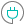#HYPGEOMDIST (PQL - xl)

This function returns the hypergeometric distribution

#### Syntax

HYPGEOMDIST(sample s, number sample, population s, number pop)

##### Function Arguments
 Name Description Type Optional sample s An integer representing the number of successes in the sample Number number sample An integer representing the size of the sample Number population s An integer representing the number of successes in the population Number number pop An integer representing the size of the population Number